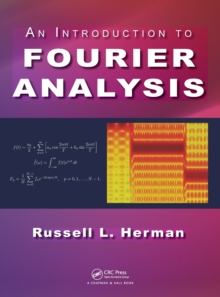Supporting your high street Find out how »
• My AccountAn Introduction to Fourier Analysis Hardback

Description

This book helps students explore Fourier analysis and its related topics, helping them appreciate why it pervades many fields of mathematics, science, and engineering. This introductory textbook was written with mathematics, science, and engineering students with a background in calculus and basic linear algebra in mind.

It can be used as a textbook for undergraduate courses in Fourier analysis or applied mathematics, which cover Fourier series, orthogonal functions, Fourier and Laplace transforms, and an introduction to complex variables.

These topics are tied together by the application of the spectral analysis of analog and discrete signals, and provide an introduction to the discrete Fourier transform.

A number of examples and exercises are provided including implementations of Maple, MATLAB, and Python for computing series expansions and transforms.

After reading this book, students will be familiar with:* Convergence and summation of infinite series* Representation of functions by infinite series* Trigonometric and Generalized Fourier series* Legendre, Bessel, gamma, and delta functions* Complex numbers and functions* Analytic functions and integration in the complex plane* Fourier and Laplace transforms. * The relationship between analog and digital signalsDr. Russell L. Herman is a professor of Mathematics and Professor of Physics at the University of North Carolina Wilmington.

A recipient of several teaching awards, he has taught introductory through graduate courses in several areas including applied mathematics, partial differential equations, mathematical physics, quantum theory, optics, cosmology, and general relativity.

His research interests include topics in nonlinear wave equations, soliton perturbation theory, fluid dynamics, relativity, chaos and dynamical systems.

Information

• Format: Hardback
• Pages: 386 pages, 4 Tables, black and white; 274 Illustrations, black and white
• Publisher: Taylor & Francis Inc
• Publication Date:
• Category: Functional analysis & transforms
• ISBN: 9781498773706

£70.99

£58.49

on all orders

Pick up orders

from local bookshops